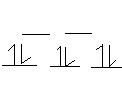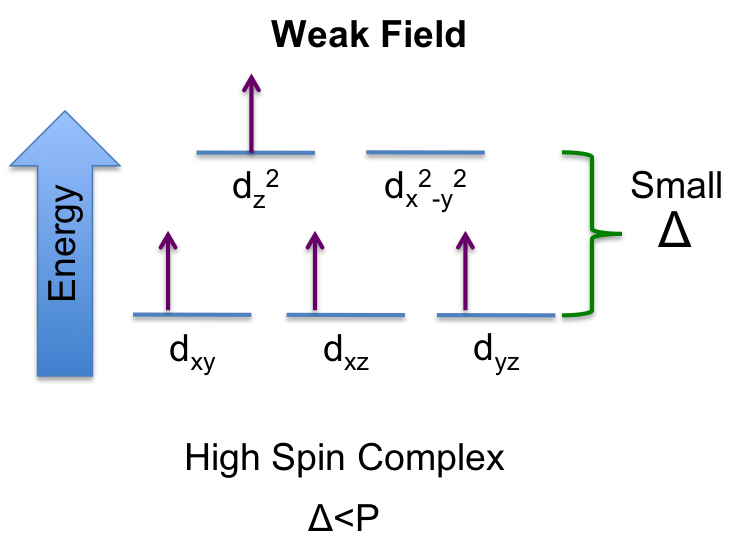# HW Solutions #5

$$\newcommand{\vecs}{\overset { \rightharpoonup} {\mathbf{#1}} }$$ $$\newcommand{\vecd}{\overset{-\!-\!\rightharpoonup}{\vphantom{a}\smash {#1}}}$$$$\newcommand{\id}{\mathrm{id}}$$ $$\newcommand{\Span}{\mathrm{span}}$$ $$\newcommand{\kernel}{\mathrm{null}\,}$$ $$\newcommand{\range}{\mathrm{range}\,}$$ $$\newcommand{\RealPart}{\mathrm{Re}}$$ $$\newcommand{\ImaginaryPart}{\mathrm{Im}}$$ $$\newcommand{\Argument}{\mathrm{Arg}}$$ $$\newcommand{\norm}{\| #1 \|}$$ $$\newcommand{\inner}{\langle #1, #2 \rangle}$$ $$\newcommand{\Span}{\mathrm{span}}$$ $$\newcommand{\id}{\mathrm{id}}$$ $$\newcommand{\Span}{\mathrm{span}}$$ $$\newcommand{\kernel}{\mathrm{null}\,}$$ $$\newcommand{\range}{\mathrm{range}\,}$$ $$\newcommand{\RealPart}{\mathrm{Re}}$$ $$\newcommand{\ImaginaryPart}{\mathrm{Im}}$$ $$\newcommand{\Argument}{\mathrm{Arg}}$$ $$\newcommand{\norm}{\| #1 \|}$$ $$\newcommand{\inner}{\langle #1, #2 \rangle}$$ $$\newcommand{\Span}{\mathrm{span}}$$$$\newcommand{\AA}{\unicode[.8,0]{x212B}}$$

14. The Co2+ ion in aqueous solution is octahedrally coordinated and paramagnetic, with three unpaired electrons. Which one or ones of the following statements follow from this observation:

1. Co(H2O)42+ is square planar
2. Co(H2O)42+ is tetrahedral
3. Co(H2O)62+ has a Δ0 that is larger than the electron-pairing energy;
4. the d levels are split in energy and filled as follows: (t2g)5(eg)2
5. the d levels are split in energy and filled as follows: (t2g)6(eg)1

- John O.

15. The coordination compound potassium hexafluorochromate(III) is paramagnetic. What is the formula for this compound? What is the configuration of the Cr d electrons?

K3[CrF6]

Fluorine is a weak field ligand in an octahedral complex. This accounts for this complex's paramagnetism.

Sean Gottlieb

16. How many unpaired electrons are there in Cr3+, Cr2+, Mn2+, Fe2+, Co3+, Co2+ in

1. a strong octahedral ligand field and
2. a very weak octahedral field?
• Cr3+ Strong Field- 3 unpaired electrons...Weak Field-3 unpaired electrons
• Cr2+ Strong Field- 2 unpaired electrons...Weak Field-4 unpaired electrons
• Mn2+ Strong Field- 1 unpaired electrons...Weak Field-5 unpaired electrons
• Fe 2+ Strong Field- 0 unpaired electrons...Weak Field-4 unpaired electrons
• Co3+ Strong Field- 0 unpaired electrons...Weak Field-4 unpaired electrons
• Co2+ Strong Field- 1 unpaired electrons...Weak Field-3 unpaired electrons

19. What is the d-orbital electronic configuration of Cr(NH3)63+? How many unpaired electrons are present? If six Br- groups were substituted for the six NH3 groups to give CrBr63-, would you expect Δ0 to increase or decrease?

Ammonia is a strong field ligand, making the complex low spin. 3 unpaired electrons are present.

Delta will decrease because Br- is a weaker field ligand than NH3

Sean Gottlieb

21. For each of the following, sketch the d-orbita1 energy levels and the distribution of d electrons among them:

1. Ni(CN)42- (square planar)

Ni2+: [Ar]3d8 CN is a strong field ligand --> low spin1. Ti(H2O)62+ (octahedral)

Ti2+: [Ar]3d2 H2O is a weak field ligand --> high spin1. NiCl42- (tetrahedra1)

Ni2+: [Ar]3d8 Cl is a weak field ligand --> high spin1. CoF63- (high-spin complex)

Co3+: [Ar]3d6 F is a weak field ligand --> high spin

6 ligands --> must be octahedral1. Co(NH3)63+ (low—spin complex)

Co3+: [Ar]3d6 NH3 is a strong field ligand --> low-spin

6 ligands --> must be octahedral23. Pt(II) can occur in the complex ion PtCl42-.

1. What is the geometry of this ion? In the valence bond theory, what Pt orbitals are used in making bonds to the Cl- ions?
2. What is the systematic name for the sodium salt of this ion?
3. Using crystal field theory, draw the d-electron configuration for this ion. ls the ion paramagnetic or diamagnetic?
4. Pt(II) can be oxidized to Pt(IV). Draw the d-electron configuration for the chloride complex ion of Pt(IV). Explain the difference between this configuration and that of Pt(II), Is the Pt(IV) chloride complex ion paramagnetic or diamagnetic?

1. square planar; d(xy) and d(x2-y2)

2. sodium tetrachloroplatinate (II)

3. According to crystal field theory, 5 d orbitals will split into three category in square planar field: d(xz), d(yz); d(z2); d(xy); d(x2-y2) (from lowest energy to highest). Pt(II) is d8, so put 8 electrons into the 5 d orbitals will result in configuration as d(xz)2, d(yz)2; d(z2)2; d(xy)2; d(x2-y2)0. Since there is no unpaired electrons, the ion is diamagnetic.

4. [Pt(IV)Cl6]2- has octahedral geometry, so 5 d orbitals will split into two category: d(xy), d(yz), d(xz); d(z2), d(x2-y2) (from lowest energy to highest). Pt(IV) is d6, so put 6 electrons into the 5 d orbitals will result in configuration as d(xy)2, d(yz)1, d(xz)1; d(z2)1, d(x2-y2)1, because Cl- is a weak field ligand. Since there are unpaired electrons, the ion is paramagnetic.

24. A solution is prepared that is 0.025M in tetraamminecopper(II), Cu(NH3)42+. What will be the concentration of Cu2+ hydrated copper ion if the ammonia concentration is 0.10, 0.50, 1.00, and 3.00 M respectively? What ammonia concentration is needed to keep the Cu2+ concentration less than 10-15 M?

[Cu(H2O)4]2+ + 4NH3 --> [Cu(NH3)4]2+ Kf = 1.1 x 1013 (from book)

[Cu(NH3)4]2+ = 0.025M Cu2+ = [Cu(H2O)4]2+

Kf = [[Cu(NH3)4]2+]/[Cu2+][NH3]4 [Cu2+] = [[Cu(NH3)4]2+]/Kf[NH3]4 = 0.025/[(1.1x1013)[NH3]4

For each given ammonia (NH3) concentration, plug it into the [Cu2+] formula to get the concentration of Cu2+ in each case:

a.) 0.10M NH3 [Cu2+] = 2.27x10-11M

b.) 0.50M NH3 [Cu2+] = 3.64x10-14M

c.) 1.00M NH3 [Cu2+] = 2.27x10-15M

d.) 3.00M NH3 [Cu2+] = 2.81x10-17M

If [Cu2+] must be below 10-15M, what must [NH3] be? Rearrange equation to solve for NH3

[NH3]= 1.23M In order for [Cu2+] to stay below 10-15M, [NH3] > 1.23M. This can be seen by looking at:

[Cu2+] = [[Cu(NH3)4]2+]/Kf[NH3]4 [NH3] is on the bottom, and as this number gets bigger, the overall right side will get smaller.

27. The ion Co(NH3)63+ is very stable, with Kf = 2.3 x 1034. If the hydrolysis constant for the ammonium ion, Kb, is 5 x 10-10, show that the equilibrium in the reaction

Co(NH3)63+ + 6H+ ↔ Co3+ + 6NH4+

lies far to the right. Then why does Co(NH3)63+ remain intact in hot concentrated sulfuric acid?

29. What is the solubility of Cu(OH)2 in pure water? In buffer at pH 6? Copper(II) forms a complex with NH3, Cu(NH3)42+, with Kf = 1.0 x 1012. What concentration of ammonia must be maintained in a solution to dissolve 0.10 mole of Cu(OH)2 per liter of solution?

Ksp (Cu(OH)2) = 2.2X10-20

In pure water, solubility is x, then Ksp = [Cu2+][OH-]2 = x(2x)2 = 2.2X10-20

solve x = 1.8X10-7 mol/L

In buffer at pH 6, [H+] = 10-6, [OH-] = 10-8

solubility is y, then Ksp = [Cu2+][OH-]2 = y(10-8)2 = 2.2X10-20

solve y = 2.2X10-4 mol/L

In Cu(NH3)42+ solution,

1/Kf = [Cu2+][NH3]4

1/(1.0X1012) = 0.1 X [NH3]4

solve [NH3] = 1.8X10-3 mol/L

31: Predict the electron configuration of an octahedral d4 complex with

d4 = 4 electrons in d-orbital

The magnitude of the crystal field splitting (Δ) determines whether the 4th electron will pair up with the electrons at the lower level or enter the upper level where it has the same electron spin as the lower level.

a. strong field ligands andIf the Δ > P (P= pairing energy: energy required to force an electron to an orbital occupied with an electron), there is a greater stability if the 4th electron is paired at the lower energy level. In this case, there are 2 unpaired electrons, which corresponds to minimum number of unpaired electrons, thus naming it low-spin. Ligands that produce large crystal field splitting leads to low-spin complex and are referred to as a strong field ligand.

b. weak field ligands, and state the number of unpaired electronsb) If Δ < P, there is a greater stability in keeping the electrons unpaired. Since there are 4 unpaired electrons when Δ < P, this corresponds to the maximum number of unpaired electrons which is referred to as high spin. Ligands that produce small Δ leads to a high-spin complex and are said to be weak field ligands.

32: Predict which of the following complexes absorbs light of the shorter wavelength and explain your reasoning: [Co(H2O)6]3+ or [Co(en)3]3+.

Both chromium(III) complexes are octahedral with an electron configuration of Cr3+ : [Ar]3d3. Using these facts, an energy level diagram of d-orbitals can be constructed.According to Hund’s Rule, the three unpaired electrons go to the lower energy d-orbital as shown in the figure below.When a photon of light is absorbed, an electron is promoted from the lower energy level to the upper energy level. The quantity of energy required for the promotion depends on the energy level separation, Δ.

According to the spectrochemical series (pg 1016 for list), “en” produces a greater crystal field splitting (Δ) of the d-orbital energy level than "H2O". Thus, [Cr(en)3]3+ will absorb light at a shorter λ (higher energy) than [Cr(H2O)6]3+.

The shorter λ = higher energy can be explained using the Planck’s Equation (E=hν) and the relationship between the speed, frequency, and wavelength of electromagnetic radiation (c=νλ) which substituting for ν gives E=hc/λ. This equation shows that wavelength is inversely related to energy.

33: Compare the magnetic properties of [Fe(H2O)6]2+ and [Fe(CN)6]4-.

34: What change in magnetic properties (if any) can be expected when NO2- ligands in an octahedral complex are replaced by Cl- ligands in a d6 complex?

The NO2- ligand is a stronger field ligand than Cl-. This translates to a greater d-orbital splitting in the NO2- complex. The Cl- complex will be in a high spin state with 4 unpaired electrons. The NO2- complex will be in a low spin state with 0 unpaired electrons. The Cl- complex is paramagnetic (total spin of 2=4*ms=1/2; remember your quantum numbers), and will therefore have appreciable magnetic interactions. The NO2- complex is diamagnetic, and will not have appreciable magnetic interactions.

35: Draw the orbital splitting diagram for the following complex and give its electron configuration: tetrahedral CoCl42-.

36: The complex ion PdCl42- is diamagnetic. Propose a structure for PdCl42-.

Pd2+ is a d8 metal ion. Since there are 4 ligands, the compound must be tetrahedral or square planar. The tetrahedral complex would yield 2 unpaired electrons. The square planar complex would yield 0 unpaired electrons. We can conclude that PdCl42- is a square planar complex.

37: Explain the following differences in color:

Observed color is really the complementary color of the absorbed color. So we can assume that the violet complex absorbs yellow light, and the yellow complex absorbs violet light. This color is due to a d-to-d transition. NH3 is a stronger field ligand than H2O. This will result in the d-to-d transition being a higher energy transition in the NH3 complex. We can therefore assume that the yellow (violet absorbing) compound is the NH3 complex. The violet (yellow absorbing) compound is the H2O complex.

The blue compound absorbs orange light. The red compound absorbs green light. Since Cl- is a weaker field ligand than H2O, the d-to-d transition should be less energetic than in the Cl- complex than in the H2O complex. Therefore, the blue (orange absorbing) compound is the Cl- complex, and the red (green absorbing) compound is the H2O complex.

CN- is a stronger field ligand than NO3-. The d-to-d transition in the CN- complex will be a higher energy transition than the NO3- complex. Therefore the Fe(CN)6 compound is yellow (violet absorbing), and the Fe(NO3)2 is green (red absorbing).

1. For [Cr(H2O)6]Cl3 and [Cr(NH3)6]Cl3, one complex is violet while the other yellow Match the expected color with each complex and explain your identifications.
2. For [Co(H2O)6]2+ and [CoCl4]2-, one complex is blue while the other reddish. Correlate a color with each complex and explain your identifications.
3. One of the following solids is yellow, and the other is green: Fe(NO3)2·6H2O versus K4[Fe(CN)6·3H2O]. Indicate which is which and explain your reasoning.

HW Solutions #5 is shared under a CC BY-NC-SA 4.0 license and was authored, remixed, and/or curated by LibreTexts.